# Introduction

The sptotal package was developed for predicting a weighted sum, most commonly a mean or total, from a finite number of sample units in a fixed geographic area. Estimating totals and means from a finite population is an important goal for both academic research and management of environmental data. One naturally turns to classical sampling methods, such as simple random sampling or stratified random sampling. Classical sampling methods depend on probability-based sample designs and are robust. Very few assumptions are required because the probability distribution for inference comes from the sample design, which is known and under our control. For design-based methods, sample plots are chosen at random, they are measured or counted, and inference is obtained from the probability of sampling those units randomly based on the design (e.g., Horwitz-Thompson estimation). As an alternative, we will use model-based methods, specifically geostatistics, to accomplish the same goals. Geostatistics does not rely on a specific sampling design. Instead, when using geostatistics, we assume the data were produced by a stochastic process with parameters that can be estimated. The relevant theory is given by Ver Hoef (2008). The sptotal package puts much of the code and plots in Ver Hoef (2008) in easily accessible, convenient functions.

In the sptotal package, our goal is to estimate some linear function of all of the sample units, call it $$\tau(\mathbf{z}) = \mathbf{b}^\prime \mathbf{z}$$, where $$\mathbf{z}$$ is a vector of the realized values for all the sample units and $$\mathbf{b}$$ is a vector of weights. By “realized,” we mean that whatever processes produced the data have already happened, and that, if we had enough resources, we could measure them all, obtaining a complete census. If $$\tau(\mathbf{z})$$ is a population total, then every element of $$\mathbf{b}$$ contains a $$1$$. Generally, $$\mathbf{b}$$ can contain any set of weights that we would like to multiply times each value in a population, and then these are summed, yielding a weighted sum.

The vector $$\mathbf{b}$$ contains the weights that we would apply if we could measure or count every observation, but, because of cost consideration, we usually only have a sample.

# Data

Prior to using the sptotal package, the data needs to be in R in the proper format. For this package, we assume that your data set is a data.frame() object, described below.

## Data Frame Structure

Data input for the sptotal package is a data.frame. The basic information required to fit a spatial linear model, and make predictions, are the response variable, covariates, the x- and y-coordinates, and a column of weights. You can envision your whole population of possible samples as a data.frame organized as follows,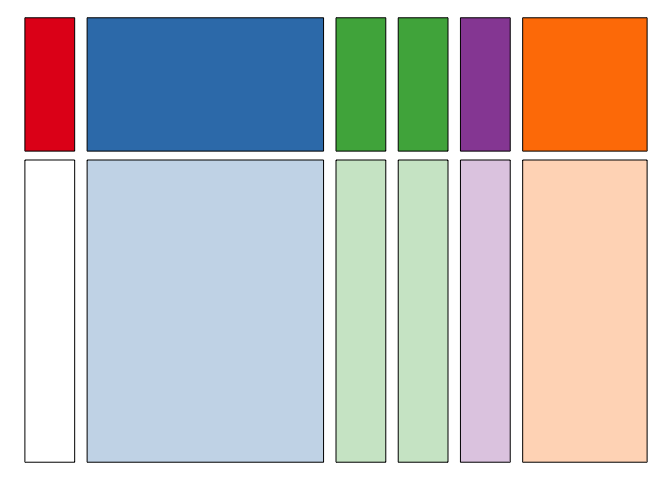where the red rectangle represents the column of the response variable, and the top part, colored in red, are observed locations, and the lower part, colored in white, are the unobserved values. To the right, colored in blue, are possibly several columns containing covariates thought to be predictive for the response value at each location. Covariates must be known for both observed and unobserved locations, and the covariates for unobserved locations are shown as pale blue below the darker blue covariates for observed locations above. It is also possible that there are no available covariates.

The data.frame must have x- and y-coordinates, and they are shown as two columns colored in green, with the coordinates for the unobserved locations shown as pale green below the darker green coordinates for the observed locations above. The data.frame can have a column of weights. If one is not provided, we assume a column of all ones so that the prediction is for the population total. The column of weights is purple, with weights for the observed locations a darker shade, above the lighter shade of purple representing weights for unsampled locations. Finally, the data.frame may contain columns that are not relevant to predicting the weighted sum. These columns are represented by the orange color, with the sampled locations a darker shade, above the unsampled locations with the lighter shade.

Of course, the data do not have to be in exactly this order, either in terms of rows or columns. Sampled and unsampled rows can be intermingled, and columns of response variable, covariates, coordinates, and weights can be also be intermingled. The figure above is an idealized graphic of the data. However, this figure helps envision how the data are used and illustrate the goal. We desire a weighted sum, where the weights (in the purple column) are multiplied with the response variable (red/white) column, and then summed. Because some of the response values are unknown (the white values in the response column), covariates and spatial information (obtained from the x- and y-coordinates) are used to predict the unobserved (white) values. The weights (purple) are then applied to both the observed response values (red), and the predicted response values (white), to obtain a weighted sum. Because we use predictions for unobserved response values, it is important to assess our uncertainty, and the software provides both an estimate of the weighted sum, mean, or total for the response variable as well as its estimated prediction variance.

## Simulated Data Creation

To demonstrate the package, we created some simulated data so they are perfectly behaved, and we know exactly how they were produced. Here, we give a brief description before using the main features of the package. To get started, install the package

install.packages("sptotal")

and then type

library(sptotal)

Type

data(simdata)

and then simdata will be available in your workspace. To see the first six observations of simdata, type

head(simdata)
#>       x     y         X1          X2         X3          X4          X5
#> 1 0.025 0.975 -0.8460525  0.11866907 -0.2123901  0.38430607  0.08154129
#> 2 0.025 0.925 -0.6583116 -0.07686491 -0.9001410 -1.24774376  1.46631630
#> 3 0.025 0.875  0.2222961 -0.22803942  0.2820468  0.20560677  0.48713665
#> 4 0.025 0.825 -0.5433925  0.56894993 -0.9839629 -0.04950434 -0.78195604
#> 5 0.025 0.775 -0.7550155 -0.72592167 -0.4217208  0.26767033  0.40493269
#> 6 0.025 0.725 -0.1786784  0.33452155 -1.2134533  2.18704575 -0.54903128
#>           X6         X7 F1 F2        Z   wts1 wts2
#> 1  1.0747592 -0.0252824  3  3 15.94380 0.0025    0
#> 2  0.1299263  1.4651052  2  5 15.04616 0.0025    0
#> 3 -0.2537515  0.2682010  2  3 14.52765 0.0025    0
#> 4 -0.3259937  0.7858140  2  5 12.13401 0.0025    0
#> 5 -1.2284475  1.2944342  2  2 11.75260 0.0025    0
#> 6 -1.0366099  0.7938890  1  4 11.58142 0.0025    0

simdata is a data frame with 400 observations. The spatial coordinates are numeric variables in columns named x and y. We created 7 continuous covariates, X1 through X7. The variables X1 through X5 were all created using the rnorm() function, so they are all standard normal variates that are independent between and within variable. Variables X6 and X7 were independent from each other, but spatially autocorrelated within, each with a variance parameter of 1, an autocorrelation range parameter of 0.2 from an exponential model, and a small nugget effect of 0.01. The variables F1 and F2 are factor variables with 3 and 5 levels, respectively. The variable Z is the response. Data were simulated from the model

\begin{align*} Z_i = 10 & + 0 \cdot X1_i + 0.1 \cdot X2_i + 0.2 \cdot X3_i + 0.3 \cdot X4_i + \\ & 0.4 \cdot X5_i + 0.4 \cdot X6_i + 0.1 \cdot X7_i + F1_i + F2_i + \delta_i + \varepsilon_i \end{align*}

where factor levels for F1 have effects $$0, 0.4, 0.8$$, and factor levels for F2 have effects $$0, 0.1, 0.2, 0.3, 0.4$$. The random errors $$\{\delta_i\}$$ are spatially autocorrelated from an exponential model,

$\textrm{cov}(\delta_i,\delta_j) = 2*\exp(-d_{i,j})$

where $$d_{i,j}$$ is Euclidean distance between locations $$i$$ and $$j$$. In geostatistics terminology, this model has a partial sill of 2 and a range of 1. The random errors $$\{\varepsilon_i\}$$ are independent with variance 0.02, and this variance is called the nugget effect. Two columns with weights are included, wts1 contains 1/400 for each row, so the weighted sum will yield a prediction of the overall mean. The column wts2 contains a 1 for 25 locations, and 0 elsewhere, so the weighted sum will be a prediction of a total in the subset of 25 locations.

The spatial locations of simdata are in a $$20 \times 20$$ grid uniformly spaced in a box with sides of length 1,

require(ggplot2)
ggplot(data = simdata, aes(x = x, y = y)) + geom_point(size = 3) +
geom_point(data = subset(simdata, wts2 == 1), colour = "red",
size = 3)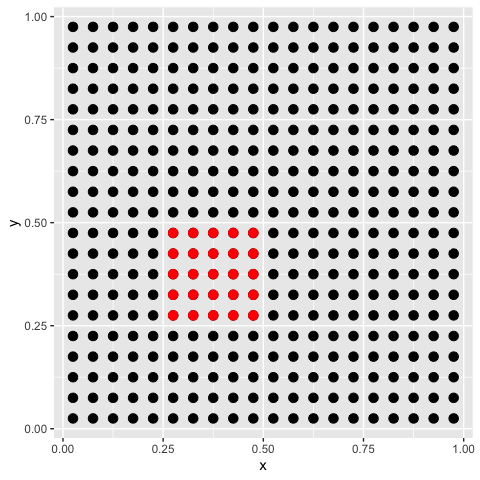The locations of the 25 sites where wts2 is equal to one are shown in red.

We have simulated the data for the whole population. This is convenient, because we know the true means and totals. In order to compare with the prediction from the sptotal package, let’s find the true population total

sum(simdata[ ,'Z'])
#>  4834.326

as well as the total in the subset of 25 sites

sum(simdata[ ,'wts2'] * simdata[ ,'Z'])
#>  273.3751

However, we will now sample from this population to provide a more realistic setting where we can measure only a part of the whole population. In order to make results reproducible, we use the set.seed command, along with sample. The code below will replace some of the response values with NA to represent the unsampled sites.

set.seed(1)
# take a random sample of 100
obsID <- sample(1:nrow(simdata), 100)
simobs <- simdata
simobs$Z <- NA simobs[obsID, 'Z'] <- simdata[obsID, 'Z'] We now have a data set where the whole population is known, simdata, and another one, simobs, where 75% of the response variable of the population has been replaced by NA. Next we show the sampled sites as solid circles, while the missing values are shown as open circles, and we use red again to show the sites within the small area of 25 locations. ggplot(data = simobs, aes(x = x, y = y)) + geom_point(shape = 1, size = 2.5, stroke = 1.5) + geom_point(data = subset(simobs, !is.na(Z)), shape = 16, size = 3.5) + geom_point(data = subset(simobs, !is.na(Z) & wts2 == 1), shape = 16, colour = "red", size = 3.5) + geom_point(data = subset(simobs, is.na(Z) & wts2 == 1), shape = 1, colour = "red", size = 2.5, stroke = 1.5)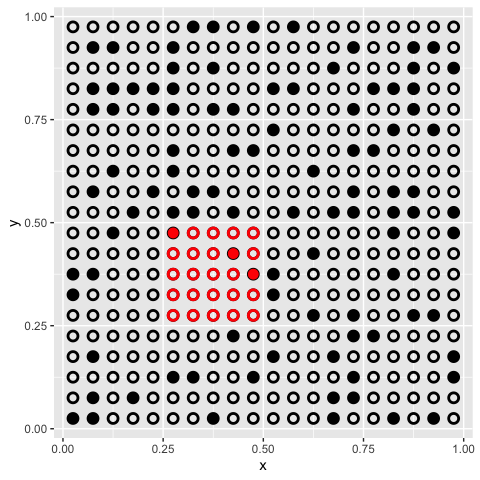We will use the simobs data to illustrate use of the sptotal package. # Using the sptotal Package After your data is in a similar format to simobs, using the sptotal package occurs in two primary stages. In the first, we fit a spatial linear model. This stage estimates spatial regression coefficients and spatial autocorrelation parameters. In the second stage, we predict the unsampled locations for the response value, and create a prediction for the weighted sum (e.g. the total) of all response variable values, both observed and predicted. To show how the package works, we demonstrate on ideal, simulated data. Then, we give a realistic example on moose data and a second example on lakes data to provide further insight and documentation. The moose example also has a section on data preparation steps. ## Fitting a Spatial Linear Model: slmfit We continue with our use of the simulated data, simobs, to illustrate fitting the spatial linear model. The spatial model-fitting function is slmfit (spatial-linear-model-fit), which uses a formula like many other model-fitting functions in R (e.g., the lm() function). To fit a basic spatial linear model we use slmfit_out1 <- slmfit(formula = Z ~ X1 + X2 + X3 + X4 + X5 + X6 + X7 + F1 + F2, data = simobs, xcoordcol = 'x', ycoordcol = 'y', CorModel = "Exponential") The documentation describes the arguments in more detail, but as mentioned earlier, the linear model includes a formula argument, and the data.frame that is being used as a data set. We also need to include which columns contain the $$x$$- and $$y$$-coordinates, which are arguments to xcoordcol and ycoordcol, respectively. In the above example, we specify 'x' and 'y' as the column coordinates arguments since the names of the coordinate columns in our simulated data set are 'x' and 'y'. We also need to specify a spatial autocorrelation model, which is given by the CorModel argument. As with many other linear model fits, we can obtain a summary of the model fit, summary(slmfit_out1) #> #> Call: #> Z ~ X1 + X2 + X3 + X4 + X5 + X6 + X7 + F1 + F2 #> #> Residuals: #> Min 1Q Median 3Q Max #> -1.9390 -0.6271 0.3338 1.2520 2.8137 #> #> Coefficients: #> Estimate Std. Error t value Pr(>|t|) #> (Intercept) 11.36965 0.60622 18.755 < 2e-16 *** #> X1 -0.05596 0.03739 -1.497 0.13812 #> X2 0.02661 0.03859 0.689 0.49241 #> X3 0.18292 0.03779 4.841 1e-05 *** #> X4 0.26487 0.03354 7.897 < 2e-16 *** #> X5 0.38434 0.03518 10.925 < 2e-16 *** #> X6 0.47612 0.06542 7.278 < 2e-16 *** #> X7 0.02893 0.06870 0.421 0.67470 #> F12 0.29596 0.08852 3.343 0.00123 ** #> F13 0.70853 0.07674 9.233 < 2e-16 *** #> F22 0.15384 0.09974 1.542 0.12664 #> F23 0.19804 0.10415 1.902 0.06057 . #> F24 0.25492 0.11697 2.179 0.03204 * #> F25 0.39748 0.13840 2.872 0.00513 ** #> --- #> Signif. codes: 0 '***' 0.001 '**' 0.01 '*' 0.05 '.' 0.1 ' ' 1 #> #> Covariance Parameters: #> Exponential Model #> Nugget 1.009265e-06 #> Partial Sill 2.930385e+00 #> Range 5.891474e-01 #> #> Generalized R-squared: 0.5996812 The output looks similar to the summary of a standard lm object, but there is some extra output at the end that gives our fitted covariance parameters. Plotting slmfit_out1 gives a semi-variogram of the residuals along with the fitted model: plot(slmfit_out1)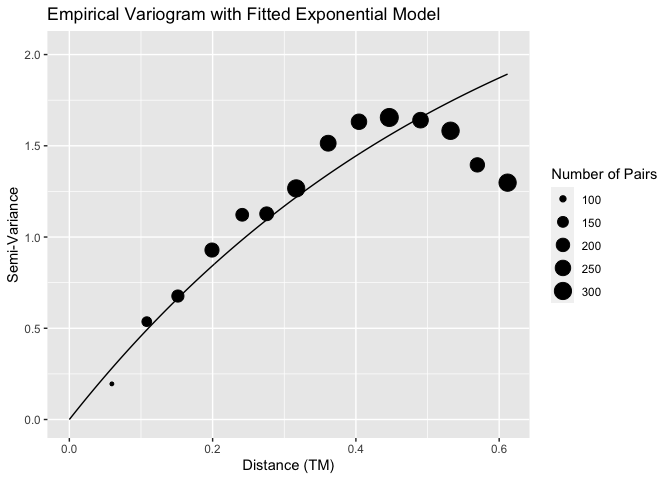Note that the fitted curve may not appear to fit the empirical variogram perfectly for a couple of reasons. First, only pairs of points that have a distance between 0 and one-half the maximum distance are shown. Second, the fitted model is estimated using REML, which may give different results than using weighted least squares. We can also examine a histogram of the residuals as well as a histogram of the cross-validation (leave-one-out) residuals: residraw <- residuals(slmfit_out1) qplot(residraw, bins = 20) + xlab("Residuals")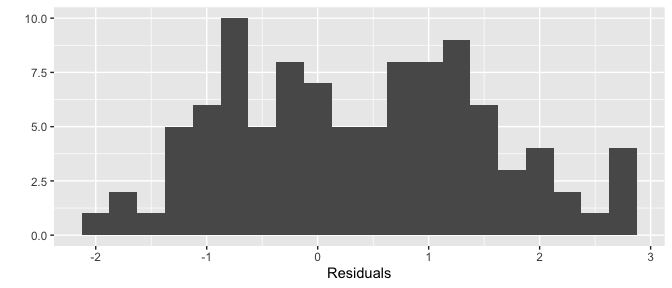residcv <- residuals(slmfit_out1, cross.validation = TRUE) qplot(residcv, bins = 20) + xlab("CV Residuals")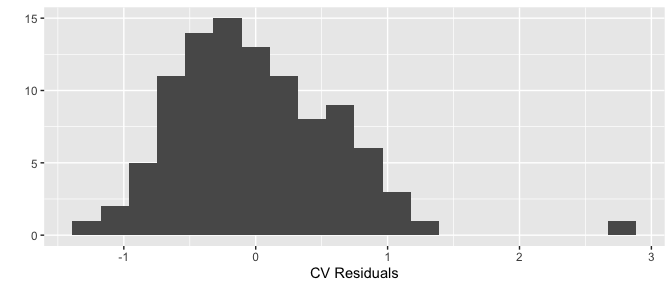There is still one somewhat large cross-validation residual for an observed count that is larger than what would be predicted from a model without that particular count. The cause of this somewhat large residual can be attributed to random chance because we know that the data was simulated to follow all assumptions. ## Prediction: predict After we have obtained a fitted spatial linear model, we can use the predict() function to construct a data frame of predictions for the unsampled sites. By default, the predict() function assumes that we are predicting the population total and outputs this predicted total, the prediction variance for the total, a 90% prediction interval for the total, and some basic summary information about the number of sites sampled, the total number of units counted, etc. We name this object pred_obj in the chunk below and also construct a 90% confidence interval for the total. pred_obj <- predict(slmfit_out1, conf_level = 0.90) pred_obj We predict a total of 4817 units in this simulated region with 90% confidence bounds of (4779, 4856). The prediction interval is fairly small because we simulated data that were highly correlated, increasing precision in prediction for unobserved sites. We can see that the prediction of the total is close to the true value of 4834.326, and the true value is within the prediction interval. To access the data.frame that was input into slmfit, but is now appended with site-by-site predictions and site-by-site prediction variances, we can use pred_obj$Pred_df. This data set might be particularly useful if you would like to generate your own map with site-by-site predictions using other tools. The site-by-site predictions for density are given by the variable name_of_response_pred_density while the site-by-site predictions for counts are given by name_of_response_pred_count. These two columns will only differ if you have provided a column for areas of each site.

moose_df$y = xy[ ,'y'] It might be helpful to compare the latitude and longitude coordinates of the original data frame to the transformed coordinates in the new data frame to make sure that the transformation seems reasonable: cbind(moose_df$x, moose_df$y, centroids$x, centroids$y) Now, the moose_df data frame is in a more workable form for the sptotal package. However, there are still a couple of issues involving how the count data is stored and which sites were sampled that may be somewhat common in real data sets, which we address next. ### Count Vector Specifications Let’s look specifically at the counts in this moose data set in the total column: head(moose_df) #> elev_mean strat surveyed census_area total x y #> 0 560.3333 L 0 0 0 38.98385 130.1806 #> 1 620.4167 L 0 0 0 34.86653 130.2284 #> 2 468.9167 L 1 0 0 30.74963 130.2815 #> 3 492.7500 L 0 0 0 26.63242 130.3400 #> 4 379.5833 L 0 0 0 22.51526 130.4038 #> 5 463.7500 L 0 0 0 38.94319 126.4665 str(moose_df$total)
#>  Factor w/ 23 levels "0","1","10","11",..: 1 1 1 1 1 1 1 1 1 1 ...

The first issue is that our original sp object had total as a factor, which R treats as a categorical variable. total should be numeric, and, in fact, the variable surveyed has the same issue. If we were to keep total as a factor and try to run slmfit, we would get a convenient error message, reminding us to make sure that our response variable is numeric, not a factor or character:

slmfit_out_moose <- slmfit(formula = total ~ strat,
data = moose_df, xcoordcol = 'x', ycoordcol = 'y',
CorModel = "Exponential")
#> Warning in stats::model.response(fullmf, "numeric"): using type = "numeric" with
#> a factor response will be ignored
#> Warning in Ops.factor(yvar, areavar): '/' not meaningful for factors
#> Error in slmfit(formula = total ~ strat, data = moose_df, xcoordcol = "x", : Check to make sure response variable is numeric, not a factor or character.

We first want to convert these two columns into numeric variables instead of factors. There are packages that can help with this conversion, like dplyr and forcats, but we opt for base R functions here.

moose_df$surveyed <- as.numeric(levels(moose_df$surveyed))[moose_df$surveyed] moose_df$total <- as.numeric(levels(moose_df$total))[moose_df$total]

This may not be an issue with the data frame you are working with.. The str() command will tell you whether your variables are coded as factors or numeric.

After conversion to numeric variables, note that the first 6 observations for the total variable are all 0, but, the first two sites and the fourth, fifth, and sixth sites weren’t actually sampled. Without some modification to this variable, sptotal wouldn’t be able to differentiate between zeroes that were zero due to a site really having 0 counts or 0 density at the site and zeroes that were zero due to the site not being sampled. The following code converts the total variable on sites that were not surveyed (surveyed = 0) to NA.

moose_df$total[moose_df$surveyed == 0] <- NA
#>   elev_mean strat surveyed census_area total        x        y
#> 0  560.3333     L        0           0    NA 38.98385 130.1806
#> 1  620.4167     L        0           0    NA 34.86653 130.2284
#> 2  468.9167     L        1           0     0 30.74963 130.2815
#> 3  492.7500     L        0           0    NA 26.63242 130.3400
#> 4  379.5833     L        0           0    NA 22.51526 130.4038
#> 5  463.7500     L        0           0    NA 38.94319 126.4665

The total column now has NA for any site that was not sampled.

### Fitting the Model and Obtaining Predictions

Now that

• we have x and y coordinates in TM format,

• our response variable is numeric and not a factor, and

• the column with our counts has NA values for sites that were not surveyed,

we can proceed to use the functions in sptotal in a similar way to how the functions were used for the simulated data. To get a sense of the data, we first give a plot of the raw observed counts:

ggplot(data = moose_df, aes(x = x, y = y)) +
geom_point(aes(colour = total), size = 4) +
scale_colour_viridis_c() +
theme_bw()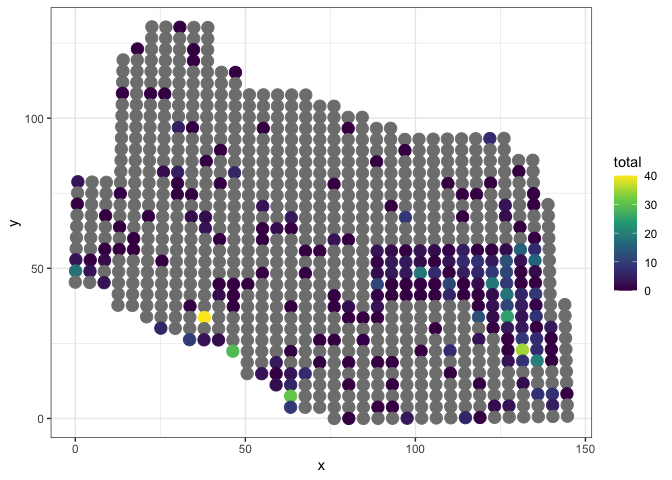where the grey circles are sites that have not been sampled.

slmfit_out_moose <- slmfit(formula = total ~ strat,
data = moose_df, xcoordcol = 'x', ycoordcol = 'y',
CorModel = "Exponential")
summary(slmfit_out_moose)
plot(slmfit_out_moose)
qplot(residuals(slmfit_out_moose, cross.validation = TRUE),
bins = 20) +
xlab("CV Residuals")

pred_moose <- predict(slmfit_out_moose)
pred_moose
plot(pred_moose)

We obtain a predicted total of 1596 animals with 90% lower and upper confidence bounds of 921 and 2271 animals, respectively. Unlike the simulation setting, there is no “true total” we can compare our prediction to, because, in reality, not all sites were sampled!

### Allowing Different Covariance Parameters for Strata

Putting strat as a predictor in the model formula means that we are allowing each stratum to have a different mean but are assuming each stratum to have the same variance and covariance. If we want to allow the two strata to have different covariance parameter estimates, we can remove strat from the model formula and add it to the stratacol argument:

slmfit_out_moose_strat <- slmfit(formula = total ~ 1,
data = moose_df, xcoordcol = 'x', ycoordcol = 'y',
stratacol = "strat",
CorModel = "Exponential")
summary(slmfit_out_moose_strat)
#> $L #> #> Call: #> total ~ 1 #> #> Residuals: #> Min 1Q Median 3Q Max #> -2.8337 -2.8337 -2.8337 0.1663 26.1663 #> #> Coefficients: #> Estimate Std. Error t value Pr(>|t|) #> [1,] 2.8337 0.3792 7.474 <2e-16 *** #> --- #> Signif. codes: 0 '***' 0.001 '**' 0.01 '*' 0.05 '.' 0.1 ' ' 1 #> #> Covariance Parameters: #> Exponential Model #> Nugget 6.548489 #> Partial Sill 23.421310 #> Range 32.274509 #> #> Generalized R-squared: 2.220446e-16 #> #>$M
#>
#> Call:
#> total ~ 1
#>
#> Residuals:
#>     Min      1Q  Median      3Q     Max
#> -4.0571 -4.0571 -2.0571  0.9429 35.9429
#>
#> Coefficients:
#>      Estimate Std. Error t value Pr(>|t|)
#> [1,]   4.0571     0.2606   15.57   <2e-16 ***
#> ---
#> Signif. codes:  0 '***' 0.001 '**' 0.01 '*' 0.05 '.' 0.1 ' ' 1
#>
#> Covariance Parameters:
#>              Exponential Model
#> Nugget                37.62337
#> Partial Sill          12.12722
#> Range                 37.68748
#>
#> Generalized R-squared: 0

There is now two sets of summary output, one for each stratum. predict() can still be used to obtain an estimate for the total (predict() also gives a predicted total for each stratum):

predict(slmfit_out_moose_strat)
#>
#> Prediction and Confidence Intervals:
#>       Prediction    SE 90% LB 90% UB
#> L         1133.4 303.2  634.6   1632
#> M          960.8 104.3  789.2   1132
#> Total     2094.2 320.7 1566.8   2622

For this example, our prediction is very different when strata are allowed separate covariance parameters (2094 moose) than when strata are forced to have the same covariance parameters (1596 moose).

To see why this is, we can examine the semi-variograms for each stratum. All functions (e.g. plot(), AIC(), coef(), etc.) that are used on an slmfit() object without stratacol specified can still be used on an slmfit() object with a stratacol specified by running the function in the following way:

plot(slmfit_out_moose_strat[])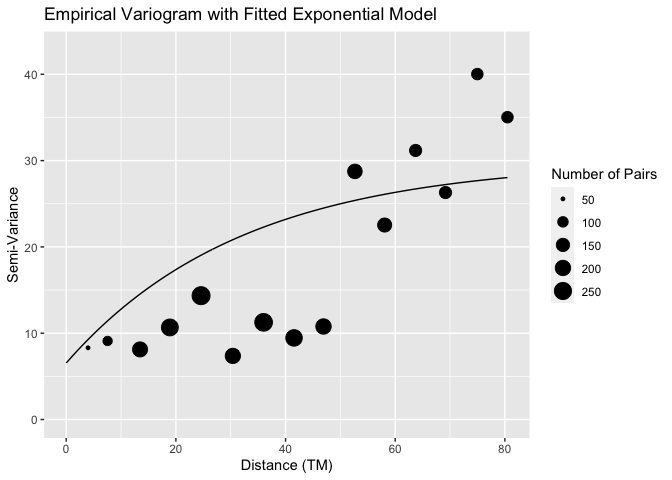plot(slmfit_out_moose_strat[])